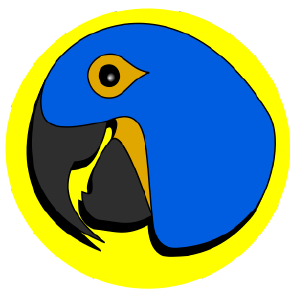blue
/
squawk
Desktop Qt based XMPP messenger https://macaw.me
You can not select more than 25 topics Topics must start with a letter or number, can include dashes ('-') and can be up to 35 characters long.

#### 73 lines 1.9 KiB Raw Blame History

 `/*` ` * Squawk messenger. ` ` * Copyright (C) 2019 Yury Gubich ` ` * ` ` * This program is free software: you can redistribute it and/or modify` ` * it under the terms of the GNU General Public License as published by` ` * the Free Software Foundation, either version 3 of the License, or` ` * (at your option) any later version.` ` * ` ` * This program is distributed in the hope that it will be useful,` ` * but WITHOUT ANY WARRANTY; without even the implied warranty of` ` * MERCHANTABILITY or FITNESS FOR A PARTICULAR PURPOSE. See the` ` * GNU General Public License for more details.` ` * ` ` * You should have received a copy of the GNU General Public License` ` * along with this program. If not, see .` ` */` `#ifndef SHARED_UTILS_H` `#define SHARED_UTILS_H` `#include ` `#include ` `#include ` `#include ` `namespace Shared {` `QString generateUUID();` `QString processMessageBody(const QString& msg);` `static const std::vector colorPalette = {` ` QColor(244, 27, 63),` ` QColor(21, 104, 156),` ` QColor(38, 156, 98),` ` QColor(247, 103, 101),` ` QColor(121, 37, 117),` ` QColor(242, 202, 33),` ` QColor(168, 22, 63),` ` QColor(35, 100, 52),` ` QColor(52, 161, 152),` ` QColor(239, 53, 111),` ` QColor(237, 234, 36),` ` QColor(153, 148, 194),` ` QColor(211, 102, 151),` ` QColor(194, 63, 118),` ` QColor(249, 149, 51),` ` QColor(244, 206, 109),` ` QColor(121, 105, 153),` ` QColor(244, 199, 30),` ` QColor(28, 112, 28),` ` QColor(172, 18, 20),` ` QColor(25, 66, 110),` ` QColor(25, 149, 104),` ` QColor(214, 148, 0),` ` QColor(203, 47, 57),` ` QColor(4, 54, 84),` ` QColor(116, 161, 97),` ` QColor(50, 68, 52),` ` QColor(237, 179, 20),` ` QColor(69, 114, 147),` ` QColor(242, 212, 31),` ` QColor(248, 19, 20),` ` QColor(84, 102, 84),` ` QColor(25, 53, 122),` ` QColor(91, 91, 109),` ` QColor(17, 17, 80),` ` QColor(54, 54, 94)` `};` `}` ```#endif // SHARED_UTILS_H ``` ``` ```